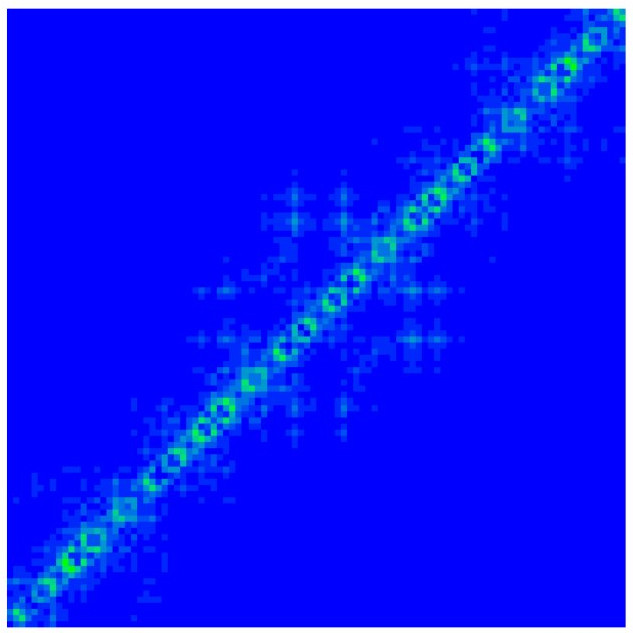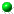Freed by interaction kinetic states
in the Harper model
by K.M.Frahm and D.L.Shepelyansky
arXiv:1509.02788[cond-mat.quant-gas]Example of delocalized FIKS eigenstate in the Harper model at lambda=2.5 and golden mean flux for Hubbard interaction U=4.5, E=-3.09 and the whole system size N=10946 (left) and zoom 100X100 of left panel bottom-left corner (right).

 ` `Article download arXiv-v1 (Sept 2015) (here); published EPJB version (here)All figs in tgz (here ) and zip (here ) formatsData Table for Fig.21 (here )Raw figs data in tgz (here ) and zip (here ) formats (see README file for explanations) This webpage is created at Sept 9, 2015 and is maintained by K.M.Frahm and D.L.Shepelyansky